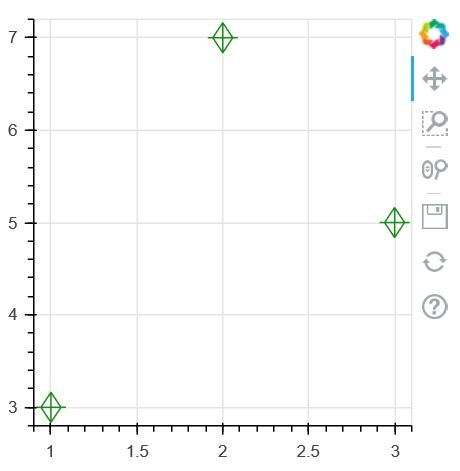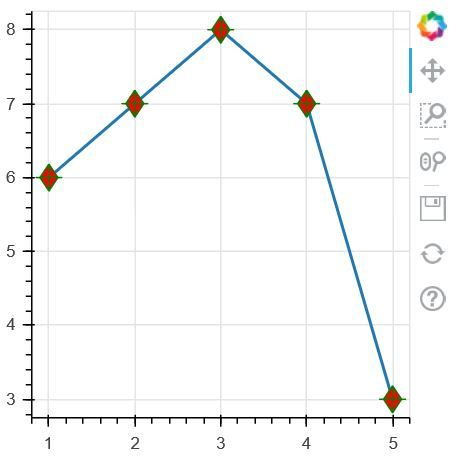# bokeh.plotting.figure.diamond_cross() function in Python

Bokeh is a data visualization library in Python that provides high-performance interactive charts and plots and the output can be obtained in various mediums like a notebook, HTML and server. Figure Class create a new Figure for plotting. It is a subclass of Plot that simplifies plot creation with default axes, grids, tools, etc.

## bokeh.plotting.figure.diamond_cross() function

The diamond_cross() function in the plotting module of the bokeh library is used to Configure and add DiamondCross glyphs to this Figure.

Syntax: diamond_cross(x, y, size=4, angle=0.0, *, angle_units=’rad’, fill_alpha=1.0, fill_color=’gray’, line_alpha=1.0, line_cap=’butt’, line_color=’black’, line_dash=[], line_dash_offset=0, line_join=’bevel’, line_width=1, name=None, tags=[], **kwargs)

Parameters: This method accept the following parameters that are described below:

• x: This parameter is the x-coordinates for the center of the markers.
• y: This parameter is the y-coordinates for the center of the markers.
• size: This parameter is the size (diameter) values for the markers in screen space units.
• angle: This parameter is the angles to rotate the markers.
• fill_color: This parameter is the fill color values for the markers.
• line_color: This parameter is the line color values for the markers with default value of black.
• line_dash: This parameter is the line dash values for the markers with default value of [].
• line_join: This parameter is the line join values for the markers with default value of bevel.
• line_width: This parameter is the line width values for the markers with default value of 1.
• name: This parameter is the user-supplied name for this model.
• tags: This parameter is the user-supplied values for this model.

Return: This method return the GlyphRenderer value.

Below examples illustrate the bokeh.plotting.figure.diamond_cross() function in bokeh.plotting:
Example 1:

## Python3

 `# Implementation of bokeh function  ` `    `  `import` `numpy as np   ` `from` `bokeh.plotting ``import` `figure, output_file, show  ` `    `  `plot ``=` `figure(plot_width ``=` `300``, plot_height ``=` `300``)  ` `plot.diamond_cross(x ``=` `[``1``, ``2``, ``3``], y ``=` `[``3``, ``7``, ``5``],   ` `            ``size ``=` `20``, fill_color ``=` `None``, ` `            ``line_color ``=` `"green"``, alpha ``=` `0.9``)  ` `    `  `show(plot)  `

Output:Example 2:

## Python3

 `# Implementation of bokeh function  ` `    `  `import` `numpy as np   ` `from` `bokeh.plotting ``import` `figure, output_file, show  ` `    `  `x ``=` `[``1``, ``2``, ``3``, ``4``, ``5``]  ` `y ``=` `[``6``, ``7``, ``8``, ``7``, ``3``]  ` `   `  `output_file(``"geeksforgeeks.html"``)  ` `   `  `p ``=` `figure(plot_width ``=` `300``, plot_height ``=` `300``)  ` ` `  `p.line(x, y, line_width ``=` `2``)  ` `p.diamond_cross(x, y, fill_color ``=``"red"``,   ` `         ``line_color ``=``"green"``, size ``=` `18``)  ` `   `  `show(p)  `

Output:Attention geek! Strengthen your foundations with the Python Programming Foundation Course and learn the basics.

To begin with, your interview preparations Enhance your Data Structures concepts with the Python DS Course.

My Personal Notes arrow_drop_upCheck out this Author's contributed articles.

If you like GeeksforGeeks and would like to contribute, you can also write an article using contribute.geeksforgeeks.org or mail your article to contribute@geeksforgeeks.org. See your article appearing on the GeeksforGeeks main page and help other Geeks.

Please Improve this article if you find anything incorrect by clicking on the "Improve Article" button below.

Article Tags :

Be the First to upvote.

Please write to us at contribute@geeksforgeeks.org to report any issue with the above content.